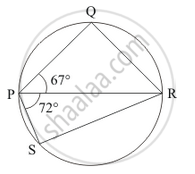Advertisement Remove all ads

# Pqrs is a Cyclic Quadrilateral Such that Pr is a Diameter of the Circle. If ∠Qpr = 67° and ∠Spr = 72°, Then ∠Qrs = - Mathematics

MCQ

PQRS is a cyclic quadrilateral such that PR is a diameter of the circle. If ∠QPR = 67° and ∠SPR = 72°, then ∠QRS =

#### Options

• 41°

•  23°

• 67°

• 18°

Advertisement Remove all ads

#### Solution

Here we have a cyclic quadrilateral PQRS with PR being a diameter of the circle. Let the centre of this circle be ‘O’.

We are given that  angleQPR  and angleSPR = 72° . This is shown in fig (2).So we see that,

$\angle QPS = \angle QPR + \angle RPS$
$= 67°+ 72°$
$= 139°$

In a cyclic quadrilateral it is known that the opposite angles are supplementary.

angleQPS + angleQRS = 180°

angleQRS = 180° - angleQPS

= 180° - 139°

= 41°

Is there an error in this question or solution?
Advertisement Remove all ads

#### APPEARS IN

RD Sharma Mathematics for Class 9
Chapter 15 Circles
Q 16 | Page 111
Advertisement Remove all ads

#### Video TutorialsVIEW ALL 

Advertisement Remove all ads
Share
Notifications

View all notifications

Forgot password?
Course